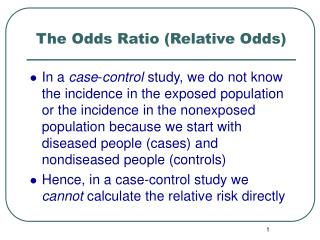DownloadDownload PresentationThe Odds Ratio (Relative Odds)

# The Odds Ratio (Relative Odds)

Télécharger la présentation## The Odds Ratio (Relative Odds)

- - - - - - - - - - - - - - - - - - - - - - - - - - - E N D - - - - - - - - - - - - - - - - - - - - - - - - - - -
##### Presentation Transcript

1. The Odds Ratio (Relative Odds) • In a case-control study, we do not know the incidence in the exposed population or the incidence in the nonexposed population because we start with diseased people (cases) and nondiseased people (controls) • Hence, in a case-control study we cannot calculate the relative risk directly

2. The Odds Ratio (Relative Odds) • Another measure of association, the odds ratio, can be obtained from either a cohort or a case-control study • The odds ratio can be used instead of the relative risk • Even though we cannot calculate a relative risk from a case-control study, under many conditions, we can obtain a very good estimate of the relative risk from a case-control study using the odds ratio

3. The Odds Ratio (Relative Odds) • In case-control studies, we have discussed the proportion of the cases who were exposed and the proportion of the controls who were exposed (Table 11-8)

4. The Odds Ratio (Relative Odds) • An alternate approach is to use the concept of odds • Suppose we are betting on a horse named Epi Beauty, which has a 60% probability of winning the race (P) • Epi Beauty therefore has a 40% probability of losing (1 - P) • If these are the probabilities, what are the odds that the horse will win the race? • The odds of an event can be defined as the ratio of the number of ways the event can occur to the number of ways the event cannot occur

5. The Odds Ratio (Relative Odds) • The odds of Epi Beauty winning, as defined above, are as follows: Probability that Epidemiology will win the race Odds = ------------------------------------------------------------- Probability that Epidemiology will lose the race P 60% = --------- = ----------- = 1.5 1 – P 40% Probability of winning = 60% Odds of winning = 1.5

6. The Odds Ratio in Cohort Studies • Fig 11-5 • What is the probability (P) that the disease will develop in an exposed person? • The answer is the incidence of the disease in the top row (exposed persons), which equals a/(a+b) • What are the odds that the disease will develop in an exposed person? • Again, looking only at the top row, the odds that the disease will develop in them are a/b

7. The Odds Ratio in Cohort Studies • Similarly, looking only at the bottom row of this table, there are c + d nonexposed persons • The probability that the disease will develop in nonexposed persons is c/(c+d) • The odds of the disease developing in these nonexposed persons are c/d

8. The Odds Ratio in Cohort Studies • In a cohort study, to answer the question of whether there is an association between the exposure and the disease • we can either use the relative risk discussed in the previous section or • we can use the odds ratio • In a cohort study, the odds ratio is defined as the ratio of the odds of development of disease in exposed persons to the odds of development of disease in nonexposed persons a/b ad ------ = --------- c/d bc

9. The Odds Ratio in a Case-Control Study • In a case-control study, we cannot calculate the relative risk directly to determine whether there is an association between the exposure and the disease • This is because, having started with cases and controls rather than with exposed and nonexposed persons, we do not have information about the incidence of disease in exposed versus nonexposed persons

10. The Odds Ratio in a Case-Control Study • However, we can use the odds ratio as a measure of the association between exposure and disease in a case-control study • But we ask different questions: "What are the odds that a case was exposed?" • Looking at the left-hand column in Table 11-8, we see that the odds of a case having been exposed are a/c • "What are the odds that a control was exposed?" • The odds of a control having been exposed are b/d

11. The Odds Ratio in a Case-Control Study • The odds ratio, in a case-control study, is defined as the ratio of the odds that the cases were exposed to the odds that the controls were exposed a/b ad ------ = --------- c/d bc • Interestingly, ad/bc represents the odds ratio in both cohort and case-control studies • In both types of studies, the odds ratio is an excellent measure of whether a certain exposure is associated with a specific disease (Fig. 11-5).